## Arcs and Chords

In Figure 1, circle O has radii OA, OB, OC and OD If chords AB and CD are of equal length, it can be shown that Δ AOB ≅ Δ DOC. This would make m ∠1 = m ∠2, which in turn would make m= m. This is stated as a theorem.Figure 1 A circle with four radii and two chords drawn.

Theorem 78: In a circle, if two chords are equal in measure, then their corresponding minor arcs are equal in measure.

The converse of this theorem is also true.

Theorem 79: In a circle, if two minor arcs are equal in measure, then their corresponding chords are equal in measure.

Example 1: Use Figure 2 to determine the following. (a) If AB = CD, and= 60°, find m CD. (b) If m=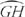and EF = 8, find GH.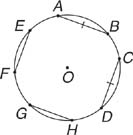Figure 2 The relationship between equality of the measures of (nondiameter) chords and equality of the measures of their corresponding minor arcs.

m= 60° (Theorem 78)

GH = 8 (Theorem 79)

Some additional theorems about chords in a circle are presented below without explanation. These theorems can be used to solve many types of problems.

Theorem 80: If a diameter is perpendicular to a chord, then it bisects the chord and its arcs.

In Figure 3UT, diameter QS is perpendicular to chord QS By Theorem 80, QR = RS, m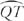= m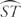, and m= m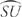.Figure 3 A diameter that is perpendicular to a chord.

Theorem 81: In a circle, if two chords are equal in measure, then they are equidistant from the center.

In Figure 4, if AB = CD, then by Theorem 81, OX = OY.Figure 4 In a circle, the relationship between two chords being equal in measure and being equidistant from the center.

Theorem 82: In a circle, if two chords are equidistant from the center of a circle, then the two chords are equal in measure.

In Figure 5, if OX = OY, then by Theorem 82, AB = CD.

Example 2: Use Figure to find x.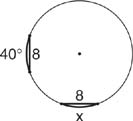Figure 5 A circle with two minor arcs equal in measure.

Example 3: Use Figure 6, in which m= 115°, m= 115°, and BD = 10, to find AC.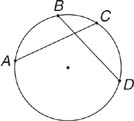Figure 6 A circle with two minor arcs equal in measure.

Example 4: Use Figure 7, in which AB = 10, OA = 13, and m ∠ AOB = 55°, to find OMm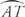and m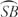.Figure 7 A circle with a diameter perpendicular to a chord.

So, ST ⊥ AB, and ST is a diameter. Theorem 80 says that AM = BM. Since AB = 10, then AM = 5. Now consider right triangle AMO. Since OA = 13 and AM = 5, OM can be found by using the Pythagorean Theorem.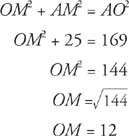Also, Theorem 80 says that m= mand m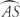= m. Since m ∠ AOB = 55°, that would make m= 55° and m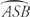= 305°. Therefore, m= 27 ½ and m= 152 ½°.

Example 5: Use Figure 8, in which AB = 8, CD = 8, and OA = 5, to find ON.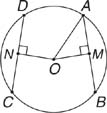Figure 8 A circle with two chords equal in measure.

By Theorem 81ON = OM. By Theorem 80AM = MB, so AM = 4. OM can now be found by the use of the Pythagorean Theorem or by recognizing a Pythagorean triple. In either case, OM = 3. Therefore, ON = 3.